# MCAT Physical : Other Electrical Principles

## Example Questions

### Example Question #1 : Other Electrical Principles

The primary coil of a transformer has 800 turns and an rms voltage of 400V. If an output voltage of 120V is needed, how many turns whould the secondary coil contain?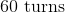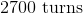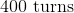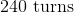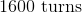Explanation:

Relevant equations: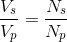For the primary coil, we have: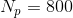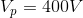And for the secondary coil: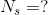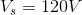Plugging these in yields: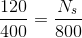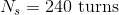### Example Question #2 : Other Electrical Principles

An electrical current is flowing through a block. It is observed that the electrical conductivity of the block is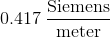, the length of the block is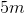and the resistance of the block is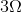. Which of the following is a valid conclusion about this block?

The block has a circular cross-section that has a radius of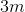The block has a square cross-section, with a height and width of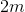The block has a circular cross-section that has a diameter ofThe block has a rectangular cross-section with a height ofand the width ofThe block has a square cross-section, with a height and width ofExplanation:

To answer this question you need to understand the relationship between electrical conductivity,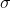, and electrical resistivity,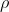: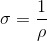This means that the electrical conductivity is the reciprocal of the electrical resistivity; therefore, the electrical resistivity of this block is: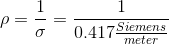Recall the definition of resistivity: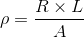Here,is the resistance,is the cross-sectional area, andis the length of the block. The question gives us resistance and length of the block, and we calculated resistivity; therefore, solving for the area of the block gives us: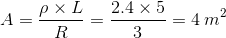The cross-sectional area of the block is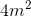.

Of the given answer choices, the only valid conclusion is that the block has a square cross-section with a height and width ofbecause this square has an area equal to the cross-sectional area of the block ().

### Example Question #3 : Other Electrical Principles

Consider a conducting rod. Which of the following is true regarding the relationship between the electrical conductivity, the resistance, and the length of the rod?

The electrical conductivity increases when both the length and the resistance of the rod increase

The electrical conductivity increases when the length of the rod increases and the resistance of the rod decreases

The electrical conductivity increases when the length of the rod decreases and the resistance of the rod increases

The electrical conductivity increases when both the length and the resistance of the rod decrease

The electrical conductivity increases when the length of the rod increases and the resistance of the rod decreases

Explanation:

The electrical conductivity is the reciprocal of electrical resistivity; therefore, an increase in electrical resistivity will lead to a decrease in electrical conductivity, and vice versa.

Recall the definition of electrical resistivity:Here,is resistance,is cross-sectional area, andis the length of the rod. This equation reveals that an increase in resistance and area will increase resistivity, whereas an increase in length will decrease resistivity. Since conductivity is the reciprocal of resistivity, increasing resistance and area will decrease conductivity, whereas increasing the length will increase conductivity.

### Example Question #4 : Other Electrical Principles

A researcher has two rods: rod A and rod B. Rod A has the same geometrical properties as rod B, but has a larger resistance. What can you conclude about the relative rates of charge flow in the two rods?

Rod A has a higher rate of charge flow than rod B because rod A has the higher electrical resistivity

Rod A has a higher rate of charge flow than rod B because rod A has the higher electrical conductivity

Rod B has a higher rate of charge flow than rod A because rod B has the higher electrical resistivity

Rod B has a higher rate of charge flow than rod A because rod B has the higher electrical conductivity

Rod B has a higher rate of charge flow than rod A because rod B has the higher electrical conductivity

Explanation:

The question is asking about the rates of charge flow. Recall that the current is defined as the amount of charge flowing through a point in a given time; therefore, we are looking for the amount of current flowing through the two rods. Current flow is higher for a material with higher electrical conductivity. This means that we need to find the rod with the higher electrical conductivity.

Since there are no easy equations to find conductivity, we need to find the resistivity first. Electrical conductivity is the reciprocal of electrical resistivity (measure of the ability of a material to resist current flow); therefore, an increase in resistivity leads to a decrease in conductivity, and vice versa. Resistivity is defined as:Here,is the resistance,is the cross-sectional area, andis the length of the rod. The question states that the two rods have the same geometrical properties; therefore, the area and the length of the rods are the same. However, the resistance of rod A is higher. This means that the resistivity is higher and conductivity, consequently, is lower for rod A. Since it has a lower conductivity, rod A has a lower charge flow rate.

### Example Question #5 : Other Electrical Principles

Which of the following is false regarding a battery?

A battery converts chemical energy into electrical energy

Recharging a battery involves a nonspontaneous chemical reaction

Electrons are reactants at an anode, and are products at a cathode

A battery houses two electrodes: the anode and the cathode

A battery converts chemical energy into electrical energy

Explanation:

Batteries are a series of electrochemical cells that use the electrical energy associated with the movement of electrons to perform other processes. The electrical energy is not itself usable; instead, batteries convert the electrical energy into other forms of energy, such as chemical energy, that can be used to drive a process. The electrical energy is derived from the movement of electrons associated with redox reactions.

Batteries contain one or more electrochemical cells. Each electrochemical cell houses a cathode and an anode, which facilitate a redox reaction. The cathode is the site of the reduction half-reaction, whereas the anode is the site of the oxidation half-reaction. Reduction is the process by which reactants gain electrons and become more negative; oxidation is the process by which reactants lose electrons and become more positive. As such, electrons are products at an anode and reactants at a cathode. The redox reaction proceeds in the spontaneous reaction direction when the battery is being used, but proceeds in the nonspontaneous reaction direction when the battery is being recharged after use. Recall that nonspontaneous reactions require energy. When you are charging a battery, you are supplying energy in the form of voltage to drive the nonspontaneous reaction. On the other hand, when you are discharging (or using) the battery, the spontaneous redox reaction is supplying energy to drive another process.

### Example Question #1 : Other Electrical Principles

In a battery, the __________ is the site of the reduction half-reaction and the __________ is the electrode where the oxidation number of the reactant increases.

anode . . . anode

anode . . . cathode

cathode . . . anode

cathode . . . cathode